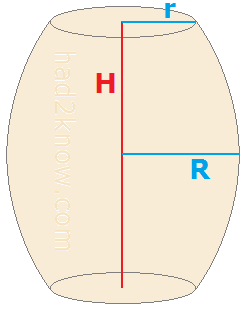# Barrel Volume Calculator

### Two Formulas for the Volume of a Barrel

 Barrel Volume Calculator Choose Equation Parabolic Simplified Height End Radius Middle Radius

A barrel is shaped much like a cylinder except that the middle bulges outward. Wine casks are traditionally barrel-shaped.

One reason why barrels are preferred for wine storage over perfect cylinders is that barrels are easier to roll in several directions on the ground. In contrast, it can be difficult to turn a corner when rolling a cylindrical container.

Also, by bulging in the middle, a convex barrel tends toward more of a spherical shape, which distributes stress more evenly.

To estimate a barrel's volume you need to know its height, end radius, and middle radius. Because the exact shape of the curve that forms a barrel's profile is unknown, the equations for barrel volume are approximations. Two such volume formulas are given below. You can also use the calculator on the left.

### Parabolic Barrel Volume FormulaIf you assume that the curve formed by the side of the barrel is a parabolic arc, then you can derive the equation for barrel volume by using integral calculus to find the volume of a solid of revolution. If the top/bottom radius is r, the middle radius R, and the height H, then the volume comes out to be

Volume = πH(3r² + 4Rr + 8R²)/15

This formula is given as the first option in the calculator above.

### Simplified Barrel Volume Formula

A more commonly used formula gives greater weight to the midradius of the barrel and has a simpler expression:

Volume = πH(r² + 2R²)/3

As you can see, this equation does not have the Rr term.

### Parabolic and Simplified Equations Compared

When R > r, the simplified formula returns a slightly larger value for the volume of a barrel. In both equations, when R = r, the equations reduce to πHr², which is the equation for the volume of a cylinder. This makes sense geometrically since the condition R = r implies there is no bulge, i.e. the sides are straight like a cylinder.

### Example of Barrel Volume Calculation

Marge has a large barrel-shaped oak wine cask. When she subtracts the thickness of the wood from her measurements, she has

H = 84 cm
R = 37 cm
r = 30 cm

Using the parabolic formula for the volume of a barrel, she estimates its volume to be

π84(3*30² + 4*37*30 + 8*37²)/15 cm³
= 318291 cm³
= 318.3 liters

If you know the volume of the barrel and two of the three dimensions (height, end radius, middle radius) then you can solve for the missing third dimension. See the Barrel Dimensions Calculator.